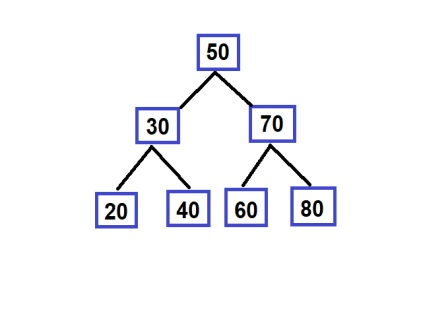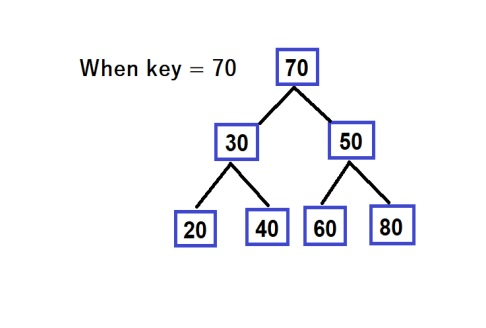# C++ Reverse a path in BST using queue

Given a binary search tree, and we are required to reverse its path from a particular key, for example.## Approach to Find the Solution

In this approach, we will make a queue and push all the nodes until we get the root.

## Example


#include <bits/stdc++.h>
using namespace std;
struct node {
int key;
struct node *left, *right;
};
struct node* newNode(int item){
struct node* temp = new node;
temp->key = item;
temp->left = temp->right = NULL;
return temp;
}
void inorder(struct node* root){
if (root != NULL) {
inorder(root->left);
cout << root->key << " ";
inorder(root->right);
}
}
void Reversing(struct node** node,
int& key, queue<int>& q1){
/* If the tree is empty then
return*/
if (node == NULL)
return;
if ((*node)->key == key){ // if we find the key
q1.push((*node)->key); // we push it into our queue
(*node)->key = q1.front(); // we change the first queue element with current
q1.pop(); // we pop the first element
}
else if (key < (*node)->key){ // if key is less than current node's value
q1.push((*node)->key); // we push the element in our queue
Reversing(&(*node)->left, key, q1); //we go to the left subtree using a recursive call
(*node)->key = q1.front(); //we reverse the elements
q1.pop(); // we pop the first element
}
else if (key > (*node)->key){ // if key greater than node key then
q1.push((*node)->key);// we push node key into queue
Reversing(&(*node)->right, key, q1);// we go to right subtree using a recursive call
(*node)->key = q1.front();// replace queue front to node key
q1.pop(); // we pop the first element
}
return;
}
struct node* insert_node(struct node* node, // function to insert node nodes in our BST
int key){
if (node == NULL)
return newNode(key); // if tree is empty we return a new node
if (key < node->key) // else we push that in our tree
node->left = insert_node(node->left, key);
else if (key > node->key)
node->right = insert_node(node->right, key);
return node; // returning the node
}
int main(){
struct node* root = NULL;
queue<int> q1;
int k = 80;
/****************Creating the BST*************************/
root = insert_node(root, 50);
insert_node(root, 30);
insert_node(root, 20);
insert_node(root, 40);
insert_node(root, 70);
insert_node(root, 60);
insert_node(root, 80);
cout << "Before Reversing :" << "\n";
inorder(root);
cout << "\n";
Reversing(&root, k, q1);
cout << "After Reversing :" << "\n";
// print inorder of reverse path tree
inorder(root);
return 0;
}

## Output

Before Reversing :
20 30 40 50 60 70 80
After Reversing :
20 30 40 80 60 70 50

## Explanation of the Above Code

In this approach, we are simply going to search for the given key. As we go through the tree, we push all the nodes in a queue, and now when we find the node with a value of the key, we swap all the path nodes’ values who queue in front, and in this process, our path becomes reversed.

## Conclusion

We solve a problem to Reverse a path in BST using a queue and using recursion. We also learned the C++ program for this problem and the complete approach (Normal) by which we solved this problem. We can write the same program in other languages such as C, java, python, and other languages. We hope you find this tutorial helpful.

Updated on: 26-Nov-2021

80 Views